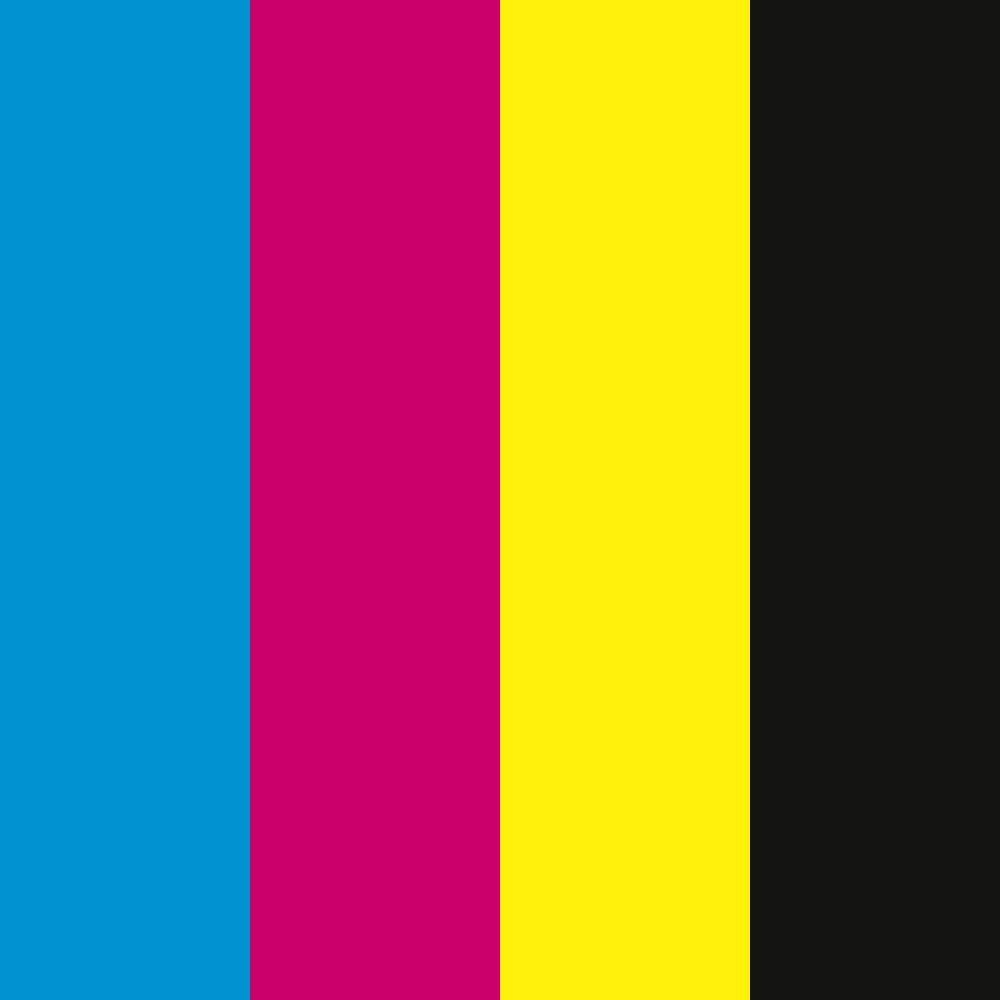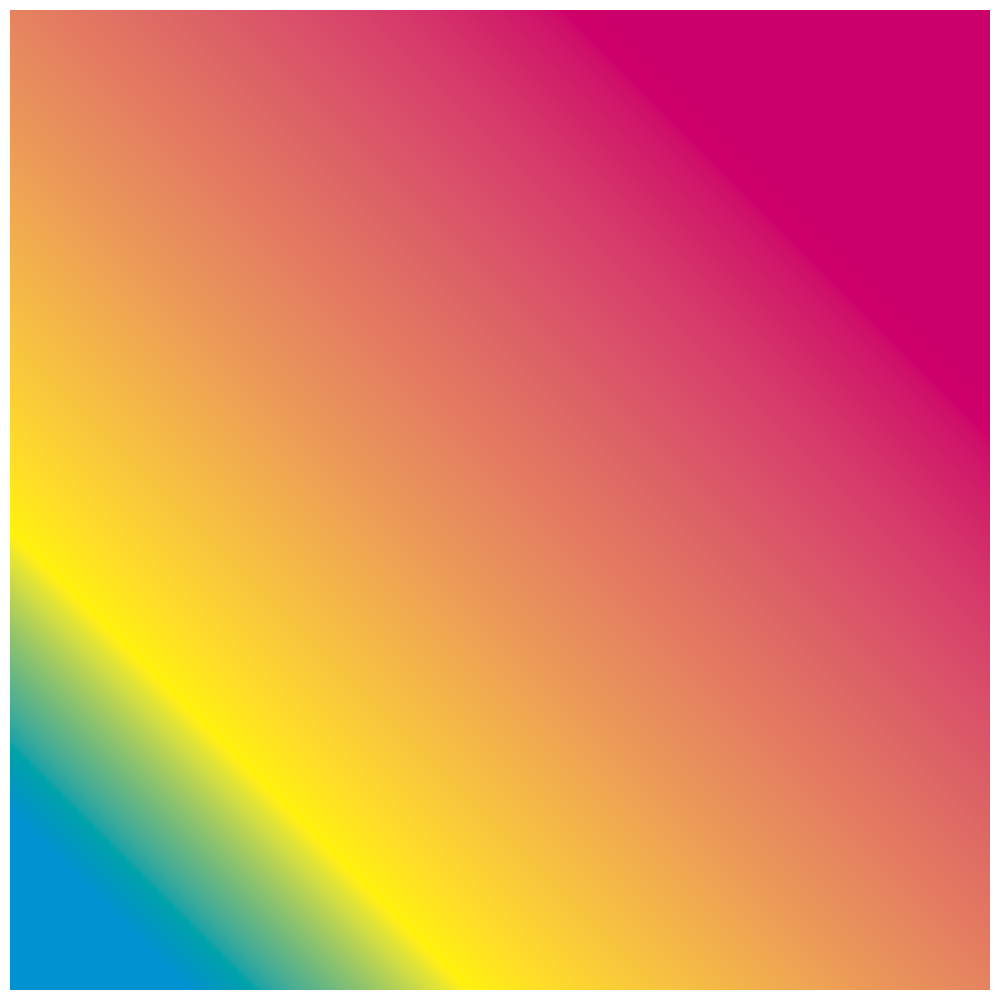# CMYK Fill¶

Set a fill before drawing a shape. A cmyk color used while exporting to a .pdf or an image. Handy if the file is used for print.

`cmykFill`(c, m=None, y=None, k=None, alpha=1)

Set a fill using a CMYK color before drawing a shape. This is handy if the file is intended for print.

Sets the CMYK fill color. Each value must be a float between 0.0 and 1.0.

```# cyan
cmykFill(1, 0, 0, 0)
rect(0, 0, 250, 1000)
# magenta
cmykFill(0, 1, 0, 0)
rect(250, 0, 250, 1000)
# yellow
cmykFill(0, 0, 1, 0)
rect(500, 0, 250, 1000)
# black
cmykFill(0, 0, 0, 1)
rect(750, 0, 250, 1000)
````cmykLinearGradient`(startPoint=None, endPoint=None, colors=None, locations=None)

A cmyk linear gradient fill with:

• startPoint as (x, y)
• endPoint as (x, y)
• colors as a list of colors, described similary as cmykFill
• locations of each color as a list of floats. (optionally)

Setting a gradient will ignore the fill.

```# set a gradient as the fill color
(100, 100),                                  # startPoint
(800, 800),                                  # endPoint
[(1, 0, 0, 0), (0, 0, 1, 0), (0, 1, 0, 0)],  # colors
[0, .2, 1]                                   # locations
)
# draw a rectangle
rect(10, 10, 980, 980)
````cmykRadialGradient`(startPoint=None, endPoint=None, colors=None, locations=None, startRadius=0, endRadius=100)

• startPoint as (x, y)
• endPoint as (x, y)
• colors as a list of colors, described similary as cmykFill
• locations of each color as a list of floats. (optionally)

Setting a gradient will ignore the fill.

```# set a gradient as the fill color
(300, 300),                                     # startPoint
(600, 600),                                     # endPoint
[(1, 0, 0, 1), (0, 0, 1, 0), (0, 1, 0, .2)],    # colors
[0, .2, 1],                                     # locations
)
# draw a rectangle
rect(10, 10, 980, 980)
````cmykShadow`(offset, blur=None, color=None)

Adds a cmyk shadow with an offset (x, y), blur and a color. The color argument must be a tuple similarly as cmykFill.

```# a cyan with some blur and a offset
cmykShadow((100, 100), 100, (1, 0, 0, 0))
# draw a rect
rect(100, 100, 600, 600)
```